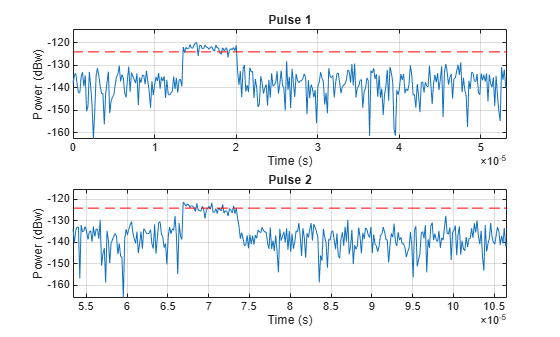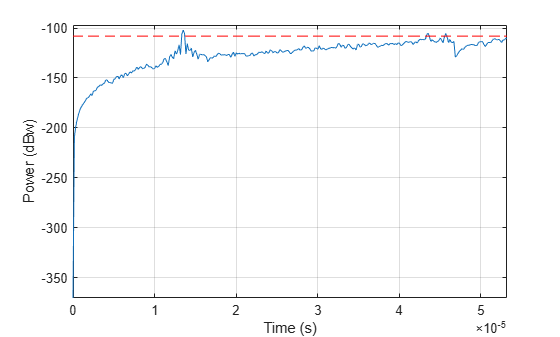Documentation

Waveform Design to Improve Performance of an Existing Radar System

This example shows how waveform type affects radar detection performance. The example considers the situation where a new performance goal is set for an existing radar system design . Since the old design can no longer achieve the desired performance, a new waveform is adopted. The example also shows how to model Swerling targets, simulate the return, and then detect the target ranges.

Design Specification Change

A monostatic pulse radar is designed in the example Designing a Basic Monostatic Pulse Radar to achieve the following goal:

1. minimum target radar cross section (RCS): 1 ${m}^{2}$, nonfluctuating;

2. maximum unambiguous range: 5 km;

3. probability of detection: 0.9;

4. probability of false alarm: 1e-6.

New Performance Requirement

After system deployment, two new requirements arise:

1. the maximum unambiguous range needs to be extended to 8 km

2. the system also needs to be able to detect Swerling case 2 targets.

Can the existing design be modified to achieve the new performance goal? To answer this question, we need to recalculate the parameters affected by these new requirements.

The first affected parameter is the pulse repetition frequency (PRF). It needs to be recalculated based on the new maximum unambiguous range.

max_range = 8000;
prf = prop_speed/(2*max_range);

Compared to the 30 kHz PRF of the existing design, the new PRF, 18.737 kHz, is smaller. Hence the pulse interval is longer. Note that this is a trivial change in the radar software and is fairly cheap in hardware cost.

waveform.PRF = prf;

Next, because the target is described using a Swerling case 2 model, we need to use Shnidman's equation, instead of Albersheim's equation, to calculate the required SNR to achieve the designated Pd and Pfa. Shnidman's equation assumes noncoherent integration and a square law detector. The number of pulses to integrate is 10.

num_pulse_int = 10;
pfa = 1e-6;
snr_min = shnidman(0.9,pfa,num_pulse_int,2)
snr_min = 6.1583

Waveform Selection

If we were to use the same rectangular waveform in the existing design, the pulse width would remain the same because it is determined by the range resolution. However, because our maximum range has increased from 5 km to 8 km and the target model switched from nonfluctuating to Swerling case 2, we need to recalculate the required peak transmit power.

lambda = prop_speed/fc;
'RCS',1,'Gain',transmitter.Gain)
peak_power = 4.4821e+04

The peak power is roughly eight times larger than the previous requirement. This is no longer a trivial modification because (1) the existing radar hardware is designed to produce a pulse with peak power of about 5200 w. Although most designs will leave some margin above the required power, it is unlikely that an existing system can accommodate eight times more power; (2) it is very expensive to replace the hardware to output such high power. Therefore, the current design needs to be modified to accommodate the new goal by using more sophisticated signal processing techniques.

Linear FM Waveform

One approach to reduce the power requirement is to use a waveform other than the rectangular waveform. For example, a linear FM waveform can use a longer pulse than a rectangular waveform. As a result, the required peak transmit power drops.

Let us examine the details of the linear FM waveform's configuration.

The desired range resolution determines the waveform bandwidth. For a linear FM waveform, the bandwidth is equal to its sweep bandwidth. However, the pulse width is no longer restricted to the reciprocal of the pulse bandwidth, so a much longer pulse width can be used. We use a pulse width that is 20 times longer and set the sample rate to be twice the pulse bandwidth.

range_res = 50;
pulse_bw = prop_speed/(2*range_res);
pulse_width = 20/pulse_bw;
fs = 2*pulse_bw;

waveform = phased.LinearFMWaveform(...
'SweepBandwidth',pulse_bw,...
'PulseWidth',pulse_width,...
'PRF',prf,...
'SampleRate',fs);

We now determine the new required transmit power needed to achieve the design requirements

'RCS',1,'Gain',transmitter.Gain)
peak_power = 2.2411e+03

This transmit power is well within the capability of our existing radar system. We have achieved a peak transmit power that can meet the new requirements without modifying the existing hardware.

transmitter.PeakPower = peak_power;

System Simulation

Now that we have defined the radar to meet the design specifications, we setup the targets and the environment to simulate the entire system.

Targets

As in the case with the aforementioned example, we assume that there are 3 targets in a free space environment. However, now the target model is Swerling case 2, the target positions and the mean radar cross sections are specified as follows:

tgtpos = [[2024.66; 0; 0],[6518.63; 0; 0],[6845.04; 0; 0]];
tgtvel = [[0;0;0],[0;0;0],[0;0;0]];
tgtmotion = phased.Platform('InitialPosition',tgtpos,'Velocity',tgtvel);

tgtrcs = [2.2 1.1 1.05];
'Model','Swerling2',...
'MeanRCS',tgtrcs,...
'OperatingFrequency',fc);

We set the seed for generating the rcs in the targets so that we can reproduce the same results.

target.SeedSource = 'Property';
target.Seed = 2007;

Propagation Environments

We also set up the propagation channel between the radar and each target.

channel = phased.FreeSpace(...
'SampleRate',waveform.SampleRate,...
'TwoWayPropagation',true,...
'OperatingFrequency',fc);

Signal Synthesis

We set the seed for the noise generation in the receiver so that we can reproduce the same results.

fast_time_grid = unigrid(0,1/fs,1/prf,'[)');
slow_time_grid = (0:num_pulse_int-1)/prf;

rxpulses = zeros(numel(fast_time_grid),num_pulse_int); % pre-allocate

for m = 1:num_pulse_int

% Update sensor and target positions
[sensorpos,sensorvel] = sensormotion(1/prf);
[tgtpos,tgtvel] = tgtmotion(1/prf);

% Calculate the target angles as seen by the sensor
[tgtrng,tgtang] = rangeangle(tgtpos,sensorpos);

% Simulate propagation of pulse in direction of targets
pulse = waveform();
[txsig,txstatus] = transmitter(pulse);
txsig = channel(txsig,sensorpos,tgtpos,sensorvel,tgtvel);

% Reflect pulse off of targets
tgtsig = target(txsig,true);

% Receive target returns at sensor
rxsig = collector(tgtsig,tgtang);
end

Range Detection

Detection Threshold

The detection threshold is calculated using the noise information, taking into consideration the pulse integration. Note that in the loaded system, as outlined in the aforementioned example, the noise bandwidth is half of the sample rate. We plot the threshold together with the first two pulses.

npower = noisepow(noise_bw,...
threshold = npower * db2pow(npwgnthresh(pfa,num_pulse_int,'noncoherent'));

pulseplotnum = 2;
fast_time_grid,slow_time_grid,pulseplotnum);The figure shows that the pulses are very wide which may result in poor range resolution. In addition, the second and third targets are completely masked by the noise.

Matched Filter

As in the case of rectangular waveform, the received pulses are first passed through a matched filter to improve the SNR. The matched filter offers a processing gain which further improves the detection threshold. In addition, the added benefit of the matched filter of a linear FM waveform is that it compresses the waveform in the time domain so that the filtered pulse becomes much narrower, which translates to better range resolution.

matchingcoeff = getMatchedFilter(waveform);
matchedfilter = phased.MatchedFilter(...
'CoefficientsSource','Property',...
'Coefficients',matchingcoeff,...
'GainOutputPort',true);
[rxpulses, mfgain] = matchedfilter(rxpulses);
threshold = threshold * db2pow(mfgain);

Compensate for the matched filter delay

matchingdelay = size(matchingcoeff,1)-1;
rxpulses = buffer(rxpulses(matchingdelay+1:end),size(rxpulses,1));

A time varying gain is then applied to the signal so that a constant threshold can be used across the entire detectable range.

range_gates = prop_speed*fast_time_grid/2;
lambda = prop_speed/fc;

tvg = phased.TimeVaryingGain(...
'RangeLoss',2*fspl(range_gates,lambda),...
'ReferenceLoss',2*fspl(max_range,lambda));
rxpulses = tvg(rxpulses);

Noncoherent Integration

We now integrate the receive pulses noncoherently to further improve the SNR. This operation is also referred to as video integration.

rxpulses = pulsint(rxpulses,'noncoherent');After video integration, the data is ready for the final detection stage. From the plot above, we see that there are no false alarms.

Range Detection

Finally, threshold detection is performed on the integrated pulses. The detection scheme identifies the peaks and then translates their positions into the ranges of the targets.

[~,range_detect] = findpeaks(rxpulses,'MinPeakHeight',sqrt(threshold));

The true ranges and the estimated ranges are

true_range = round(tgtrng)
true_range = 1×3

2025        6519        6845

range_estimates = round(range_gates(range_detect))
range_estimates = 1×3

2025        6525        6850

Note that these range estimates are only accurate up to the range resolution that can be achieved by the radar system, which is 50 m in this example.

Summary

In this example, we used the chirp waveform for range detection. By using the chirp waveform, we were able to reduce the required peak transmit power, thus achieving a larger detectable range of 8km for Swerling case 2 targets.